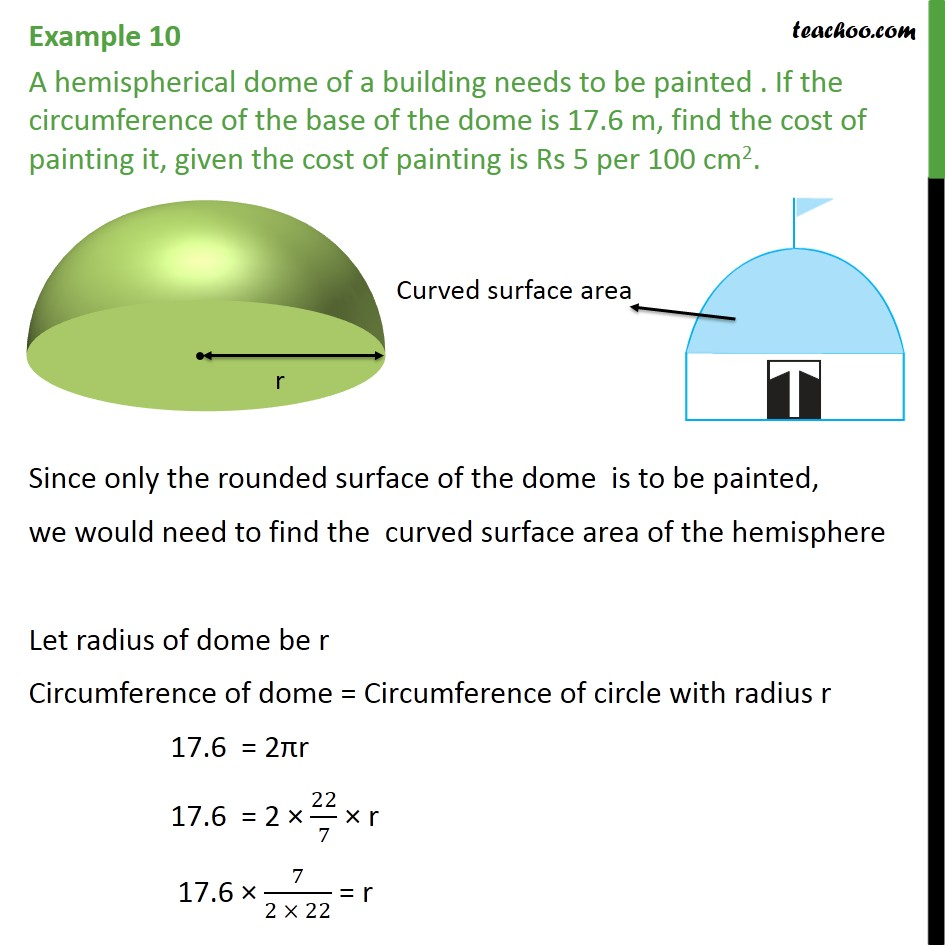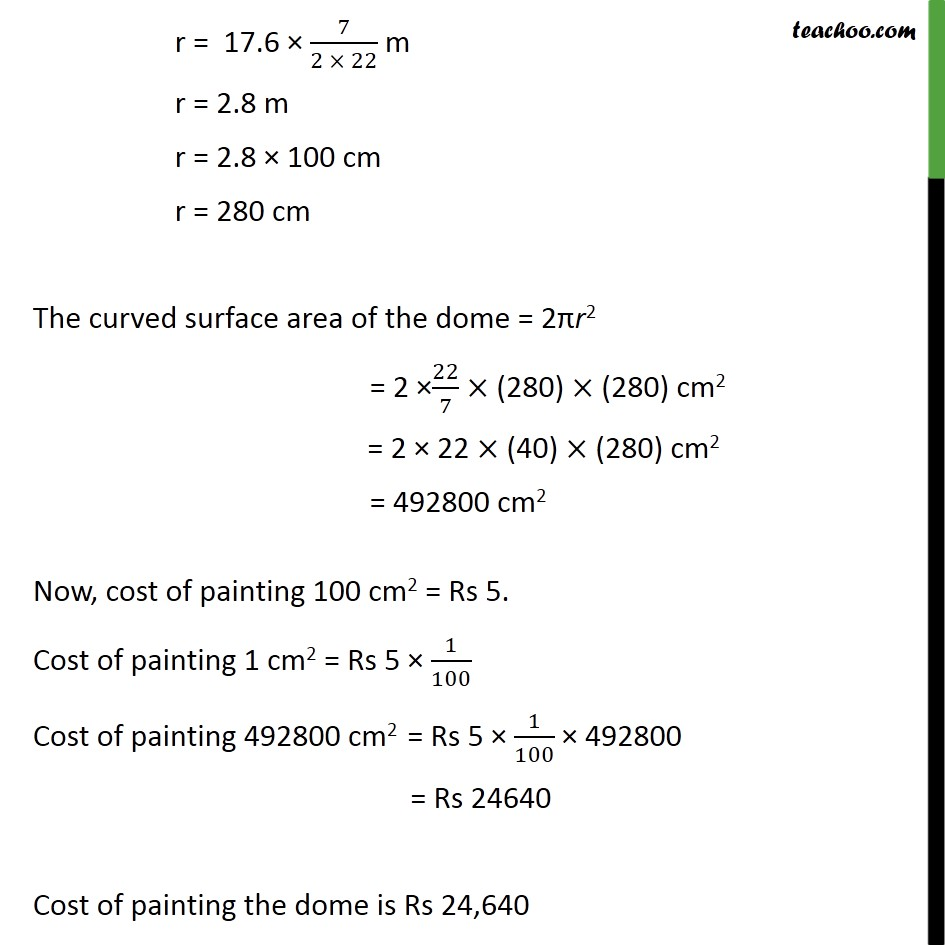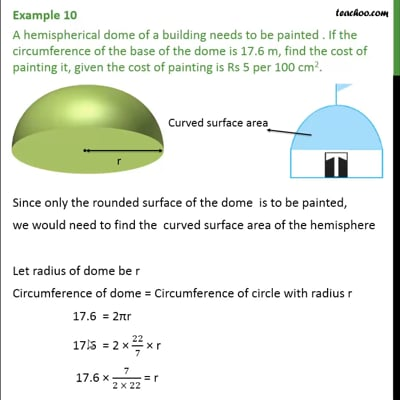Area Of Hemisphere

Chapter 11 Class 9 Surface Areas and Volumes
Concept wiseThis video is only available for Teachoo black users

Learn in your speed, with individual attention - Teachoo Maths 1-on-1 Class

### Transcript

Example 7 A hemispherical dome of a building needs to be painted . If the circumference of the base of the dome is 17.6 m, find the cost of painting it, given the cost of painting is Rs 5 per 100 cm2. Since only the rounded surface of the dome is to be painted, we would need to find the curved surface area of the hemisphere Let radius of dome be r Circumference of dome = Circumference of circle with radius r 17.6 = 2πr 17.6 = 2 × 22/7 × r 17.6 × 7/(2 × 22) = r r = 17.6 × 7/(2 × 22) m r = 2.8 m r = 2.8 × 100 cm r = 280 cm The curved surface area of the dome = 2πr2 = 2 ×22/7 × (280) × (280) cm2 = 2 × 22 × (40) × (280) cm2 = 492800 cm2 Now, cost of painting 100 cm2 = Rs 5. Cost of painting 1 cm2 = Rs 5 × 1/100 Cost of painting 492800 cm2 = Rs 5 × 1/100 × 492800 = Rs 24640 Cost of painting the dome is Rs 24,640